Next: Finding the root of Up: The Trust-Region subproblem Previous: must be positive definite.   Contents

Subsections

# Explanation of the Hard case.

Theorem 2 tells us that we should be looking for solutions to 4.9 and implicitly tells us what value of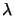we need. Suppose thathas an eigendecomposition: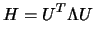(4.8)

where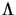is a diagonal matrix of eigenvalues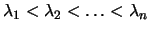and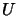is an orthonormal matrix of associated eigenvectors. Then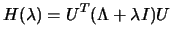(4.9)

We deduce immediately from Theorem 2 that the value ofwe seek must satisfy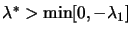(as only then is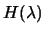positive semidefinite) (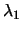is the least eigenvalue of). We can compute a solutionfor a given value ofusing: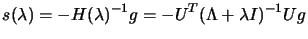(4.10)

The solution we are looking for depends on the non-linear inequality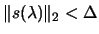. To say more we need to examine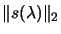in detail. For convenience we define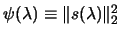. We have that: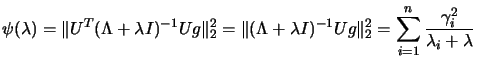(4.11)

where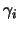is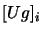, the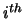component of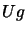.

## Convex example.

Suppose the problem is defined by: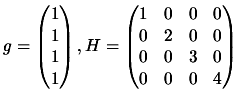We plot the function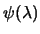in Figure 4.1. Note the pole ofat the negatives of each eigenvalues of. In view of theorem 2, we are only interested in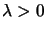. If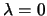, the optimum lies inside the trust region boundary. Looking at the figure, we obtain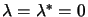, for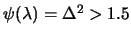. So, it means that if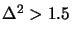, we have an internal optimum which can be computed using 4.9. If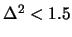, there is a unique value of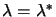(given in the figure and by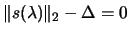(4.12)

which, used inside 4.9, give the optimal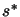.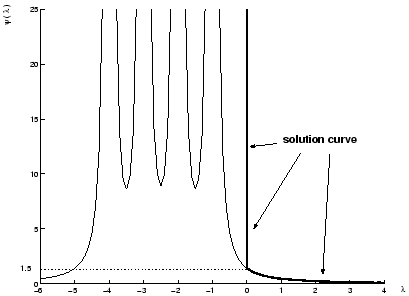## Non-Convex example.

Suppose the problem is defined by: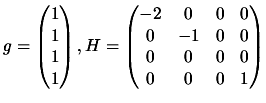We plot the functionin Figure 4.2. Recall thatis defined as the least eigenvalue of. We are only interested in values of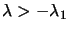, that is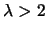. For value of, we haveNOT positive definite. This is forbidden due to theorem 2. We can see that for any value of, there is a corresponding value of. Geometrically, H is indefinite, so the model function is unbounded from below. Thus the solution lies on the trust-region boundary. For a given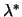, found using 4.14, we obtain the optimalusing 4.9.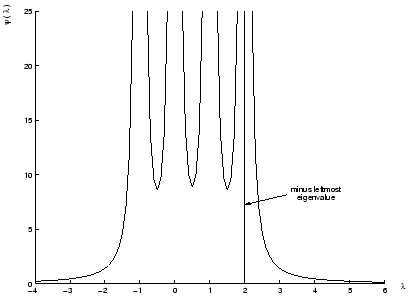## The hard case.

Suppose the problem is defined by: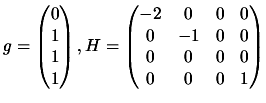We plot the functionin Figure 4.3. Again,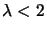, is forbidden due to theorem 2. If,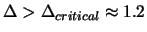, there is no acceptable value of. This difficulty can only arise whenis orthogonal to the space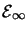, of eigenvectors corresponding to the most negative eigenvalue of. When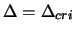, then equation 4.9 has a limiting solution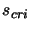, where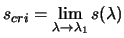.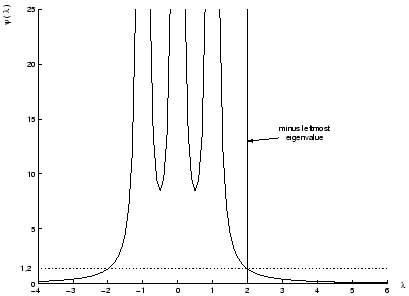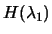is positive semi-definite and singular and therefore 4.9 has several solutions. In particular, if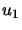is an eigenvector corresponding to, we have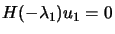, and thus: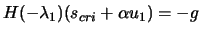(4.13)

holds for any value of the scalar. The value ofcan be chosen so that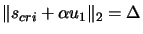. There are two roots to this equation: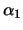and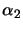. We evaluate the model at these two points and choose as solution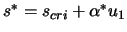, the lowest one.Next: Finding the root of Up: The Trust-Region subproblem Previous: must be positive definite.   Contents
Frank Vanden Berghen 2004-04-19